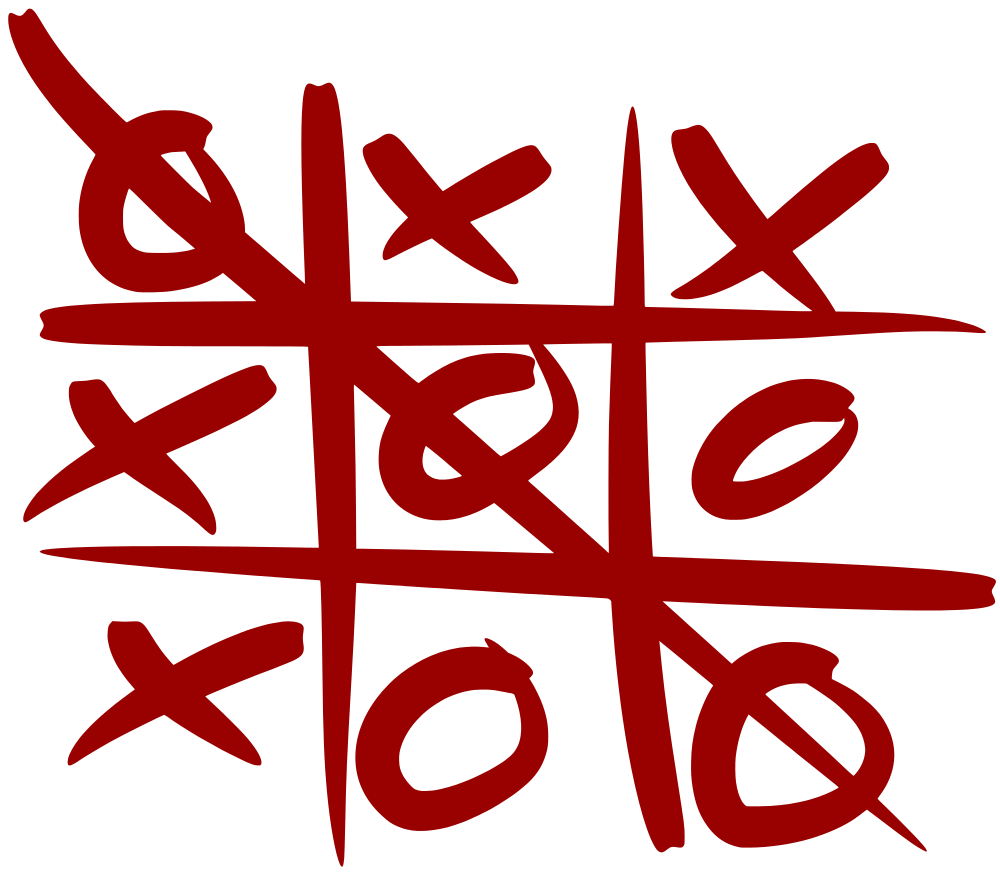# Tic-Tac-Tutorial: Introduction

We stare at a blank slate. An empty project.

Where do we go from here?

I usually start by identifying the core concepts and the entities, and start creating classes. For me, thinking about how things are conceptually defined and related helps to flesh out the big picture.Let’s begin by listing the things that make up a Tic-Tac-Toe game:

• 2 players
• A game
• The grid
• Game moves
• Move rules
• End-game rules/conditions

That’s a good start. We’ve identified all the entities (players, game, grid, moves) as well as the critical logic (move rules, end-game rules).

Let’s start writing classes. We’ll worry about a clean design later, for now let’s just brainstorm.
(BTW I’m purposefully making it an imperfect design so that we can fix it later).

```    public class Player
{
/// <summary>
/// X, O, or uninitialized
/// </summary>
public char? Mark { get; set; }

/// <summary>
/// The player's name
/// </summary>
public string Name { get; set; }
}
```

OK, we’ve got a player. Well, a game needs 2 of them, right? What else can we define about a Game of TicTacToe?

```    public class Game
{
/// <summary>
/// The X player
/// </summary>
public Player Player1 { get; set; }

/// <summary>
/// The O player
/// </summary>
public Player Player2 { get; set; }

/// <summary>
/// The name of the player whose move it is
/// </summary>
public string PlayerTurn { get; set; }

/// <summary>
/// Whether or not the game is finished
/// </summary>
public bool IsFinished { get; set; }

/// <summary>
/// Whether or not the game is a draw
/// </summary>
public bool IsDraw { get; set; }

/// <summary>
/// The name of the player that won the game
/// </summary>
public string Winner { get; set; }

/// <summary>
/// The game board
/// </summary>
public Board Board { get; set; }
}
```

That looks like a game! Hmm how are we going to represent the game grid, though…

```    public class Board
{
/// <summary>
/// The mark of the player who owns square 1 (top left)
/// </summary>
public char? Square1 { get; set; }

/// <summary>
/// The mark of the player who owns square 2 (top middle)
/// </summary>
public char? Square2 { get; set; }

/// <summary>
/// The mark of the player who owns square 3 (top right)
/// </summary>
public char? Square3 { get; set; }

/// <summary>
/// The mark of the player who owns square 4 (center left)
/// </summary>
public char? Square4 { get; set; }

/// <summary>
/// The mark of the player who owns square 5 (center middle)
/// </summary>
public char? Square5 { get; set; }

/// <summary>
/// The mark of the player who owns square 6 (center right)
/// </summary>
public char? Square6 { get; set; }

/// <summary>
/// The mark of the player who owns square 7 (bottom left)
/// </summary>
public char? Square7 { get; set; }

/// <summary>
/// The mark of the player who owns square 8 (bottom middle)
/// </summary>
public char? Square8 { get; set; }

/// <summary>
/// The mark of the player who owns square 9 (bottom right)
/// </summary>
public char? Square9 { get; set; }
}
```

Hmm, that’s a little clunky, but we’ll stick with it for now.

We’ve defined all the objects we think we need at this point, which is a great start. We haven’t yet defined any logic to actually run the game, which is fine, because we’ll want to start writing tests first.

Let’s figure out what logic we’ll need:

1. Making Moves
1.1. The current player can put their mark into any square that is unoccupied
1.2. The current player cannot put their mark into a square that is occupied
2. End game
2.1. After every move, evaluate whether or not there is a winner
2.1.1. Check for the same mark in 8 ways: top across, middle across, bottom across, left down, center down, right down, diagonal right, diagonal left
2.2. if there is a winner, then update the fields and prevent further moves
2.3. if there is no winner and all the squares are taken, then update the fields and prevent further moves

That should cover it – but, WE STILL MUST NOT WRITE CODE… yet.

To be continued…